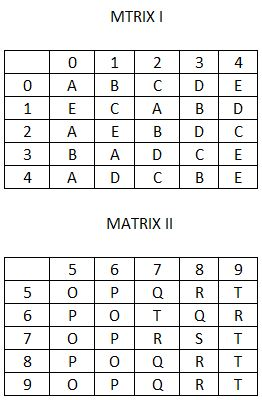Question 200

# A word is represented by only one set of numbers as given in any one of the alternatives. The sets of numbers given in the alternatives are represented by two classes of alphabets as in two matrices given below. The columns and rows of Matrix I are numbered from 0 to 4 and that of Matrix II are numbered from 5 to 9 A letter from these matrices can be represented first by its row and next by its column, e.g., ‘A’ can be represented by 00, 12 etc. and ‘V can be represented by 56, 76 etc. Similarly, you have to identify the set for the word ‘PARROT.Solution

P can be 56 or 65 or 76 or 85 or 96
A can be 00 or 20 or 40 or 31 or 12
R can be 77 or 58 or 88 or 98 or 69
R can be 77 or 58 or 88 or 98 or 69
O can be 55 or 66 or 75 or 86
T can be 59 or 67 or 79 or 89 or 99
Hence, 56, 00, 77, 88, 86, 99

• Free SSC Study Material - 18000 Questions
• 230+ SSC previous papers with solutions PDF